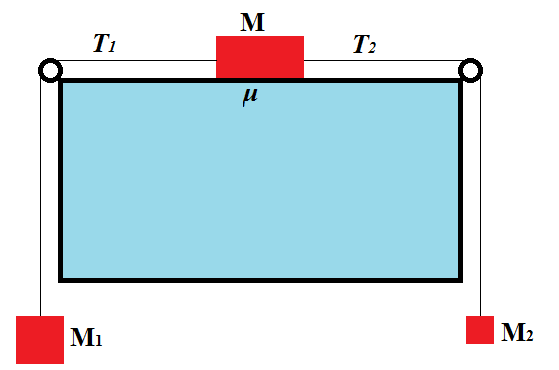## Thursday, June 7, 2018

### Unizor - Physics4Teens - Mechanics - Dynamics - Friction - Problems 3

Notes to a video lecture on http://www.unizor.com

Friction Problems 3

Problem A

An object of mass M can slide on a horizontal table. Two weights of masses M1 and M2 are attached to it on both sides of a table with weightless thread as on this picture.Assuming mass M1 is greater than M2, the object will start sliding to the left under a pull of a bigger weight.

Tensions T1 and T2, as well as friction act on an object on a table.

Assume that this object, as a result of actions of these forces, moves with acceleration a.

What is the friction coefficient μ and magnitudes of tensions T1 and T2?

Hint:

Use the Second Newton's Law for each of three objects (one on a table and two weights) participating in the motion.

Solution:

For object on a table:

T1 − T2 − μ·M·g = M·a

For weight on the left:

M1·g − T1 = M1·a

For weight on the right:

T2 − M2·g = M2·a

Got linear system of three equations with three unknowns: friction coefficient μ and magnitudes of tensions T1 and T2.

## Friday, June 1, 2018

### Unizor - Physics4Teens - Mechanics - Dynamics - Friction - Problems 2

Notes to a video lecture on http://www.unizor.com

Friction Problems 2

Problem A

There is a ramp of mass M and angle φ to horizon, lying, but not fixed, on a horizontal surface (it's like a triangular prism lying on a side).

An object of mass m slides down this ramp. As it slides, the ramp also moves along the horizontal surface it's lying on.

What is the acceleration of a ramp relative to a horizontal surface, as
object slides down, if the coefficient of friction between an object and
a ramp is μ0, and the coefficient of friction between a ramp and a horizontal surface is μ1?

The free fall acceleration is g, so the weight of an object of mass m is m·g.

Hint:

(1) Review Problem B from Mechanics - Dynamics - Superposition of Forces - Inclined Plane of this course.

(2) Take into account that, according to the Third Newton's Law,
friction between an object and a ramp is, on one hand, a force exhorted
by a ramp that pulls object uphill against its sliding downhill and, on
the other hand, is an action of an object on the ramp in an opposite
direction, slowing its horizontal movement.